# Vibration-Rotation Spectrum of HClA classic among molecular spectra, the infrared absorption spectrum of HCl can be analyzed to gain information about both rotation and vibration of the molecule.

The absorption lines shown involve transitions from the ground to first excited vibrational state of HCl, but also involve changes in the rotational state. The rotational angular momentum changes by 1 during such transitions. If you had a transition from j=0 in the ground vibrational state to j=0 in the first excited state, it would produce a line at the vibrational transition energy. As observed, you get a closely spaced series of lines going upward and downward from that vibrational level difference. The splitting of the lines shows the difference in rotational inertia of the two chlorine isotopes Cl-35(75.5%) and Cl-37(24.5%).

From the spectrum above, you can examine details about the following:

 Bond force constant Bond length j for peak intensity Relative intensities
 Pure rotational transitions in HCl
 Vibration-rotation spectrum of HBr
Index

Molecular spectra concepts

Reference
Tipler & Llewellyn
Sec. 9-4

 HyperPhysics***** Quantum Physics R Nave
Go Back

# Bond Force Constant for HClBy treating the vibrational transition in the HCl spectrum from its ground to first excited state as a quantum harmonic oscillator, the bond force constant can be calculated. This transition frequency is related to the molecular parameters by: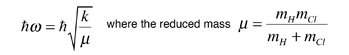The desired transition frequency does not show up directly in the observed spectrum, because there is no j=0, v=0 to j=0, v=1 transition; the rotational quantum number must change by one unit. It can be approximated by the midpoint between the j=1,v=0->j=0,v=1 transition and the j=0,v=0->j=1,v=1 transition. This assumes that the difference between the j=0 and j=1 levels is the same for the ground and first excited state, which amounts to assuming that the first excited vibrational state does not stretch the bond. This "rigid-rotor" model can't be exactly correct, so it introduces some error. For the HCl molecule, the needed reduced mass is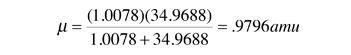Note that this is almost just the mass of the hydrogen. The chlorine is so massive that it moves very little while the hydrogen bounces back and forth like a ball on a rubber band! Substituting the midpoint frequency into the expression containing the bond force constant gives:Despite the approximations, this value is quite close to the value given in the table.

 Pure rotational transitions in HCl
 Example bond force constants
Index

Molecular spectra concepts

Reference
Tipler & Llewellyn
Sec. 9-4

 HyperPhysics***** Quantum Physics R Nave
Go Back

# Bond Length of HClA bond length for the HCl molecule can be calculated from the HCl spectrum by assuming that it is a rigid rotor and solving the Schrodinger equation for that rotor. For a free diatomic molecule the Hamiltonian can be anticipated from the classical rotational kinetic energy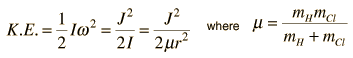and the energy eigenvalues can be anticipated from the nature of angular momentum.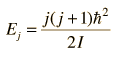Assuming that the bond length is the same for the ground and first excited states, the difference between the j=1,v=0->j=0,v=1 transition and the j=0,v=0->j=1,v=1 transition frequencies can be used to estimate the bond length. The separation between the two illustrated vibration-rotation transitions is assumed to be twice the rotational energy change from j=0 to j=1.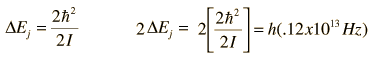Substitution of numerical values leads to an estimate of the bond length r:This compares reasonably with the value r=.127 nm obtained from pure rotational spectra.

 Pure rotational transitions in HCl
 Table of diatomic bond lengths
Index

Molecular spectra concepts

 HyperPhysics***** Quantum Physics R Nave
Go Back